# Simulating DNA Sequences

In this tutorial, you will develop an intuition for continuous-time Markov models of the sort used by RevBayes, as well as by other programs, such as PAUP, MrBayes, PhyloBayes, and others. The basic phylogenetic model, used in all of these programs has several components. You are probably familiar with two of them: a phylogenetic tree describing the relationships among the species with the branch lengths specified in terms of expected amount of change. The difficult part of the phylogenetic model is how character change is modeled along the branches of the tree. All of these programs assume that characters evolve along the branches of the tree according to a continuous-time Markov model.

## Generating uniform and exponential random variables

If you had a highschool social life as awkward as this author’s, you already own a 10-sided die (d10, in the gaming lingo). If you actually had friends in high school, however, you may need to buy such a die. Go to a gaming store and tell them that you want to buy a “d10.” Alternatively, buy a 10-sided die from Amazon.

Examine your new die. Note that it has ten faces, with each face numbered from 0 to 9. You can generate a random number on the interval (0,1) by repeatedly rolling your die. You will assume the “0.” of the number and use the die to randomly generate the digit in the tenths place (0._), hundreths place (0._ _), thousandths place (0. _ _ _) etc. until you have the random number to the desired precision. Let’s try it! I rolled my die three times and saw, in order, the numbers 0, 4, and 7. My uniform(0,1) random number, then, is $u = 0.047$. Try it yourself. With three rolls of the die, you can generate a random number to a precision of three decimal places. Do you understand why the number you generate in this manner is uniformly-distributed on the interval (0,1)? For reference, the uniform(0,1) probability distribution looks like,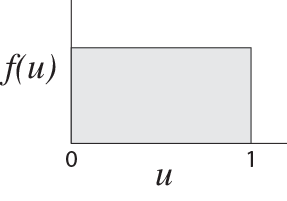and is sometimes referred to as the rectangular distribution, for obvious reasons.

The uniform distribution is only one of many distributions. You have probably heard of at least some of the more common distributions (the normal, log-normal, binomial, gamma, Poisson, exponential, $\chi^2$, Student’s t) and perhaps you’ve heard of some of the more obscure distributions, too (Wishart, Normal inverse Wishart, and Weibull, among others). When simulating DNA sequence evolution on a tree, in addition to the uniform(0,1) random numbers, we will need to generate exponential random variables.

The exponential distribution is used to model waiting times. Imagine that something occurs at a constant rate, $\lambda$. The time until that something occurs is exponentially distributed with parameter $\lambda$. The exponential distribution looks like,While we can use the die to generate a uniformly-distributed random number, we cannot directly generate an exponentially-distributed number. That said, we can generate an exponential random variable from our uniformly-distributed random number using some math. First, generate a uniform(0,1) random number using your die, called $u$. We can convert this uniform(0,1) random number to an exponential random number using the following equation: $t = -{\log(u) \over \lambda}$ where $\lambda$ is the rate at which something occurs and $\log$ is the natural log function. (You can access the natural log function on your smart phone by going to the calculator app and turning the phone on its side, thereby revealing the full functionality of the calculator.) The variable $t$ is exponentially distributed.

Let’s try it. We will generate an exponential random number when the rate parameter is $\lambda = 10$. With my die, I generated a uniform(0,1) random number: $u = 0.948$. Using my calculator, I convert it to an exponential random number: $t = -{\log(0.948) \over 10} = 0.00534$

## The parameters for the simulation

We now have the machinery needed to generate uniform and exponential random numbers. For the simulation of DNA sequences on a tree, however, we need to choose some simulation parameters. Specifically, we need the tree topology, branch lengths, and rate matrix of the continuous-time Markov model that describes how the DNA sequences change over time.

We will assume the following tree for the simulations: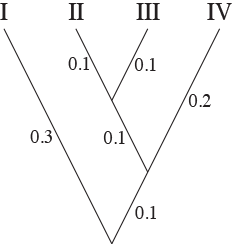We will simulate on this tree for no particular reason except that I like this tree. Note the branch lengths on the tree. The branch lengths are in terms of expected number of substitutions per site. Again, the branch lengths were an arbitrary choice that I made.

The last part of the model that must be specified is the rate matrix of the continuous-time Markov process that describes how the DNA sequences change on the tree. We will assume that sequences evolve according to the HKY85 model of DNA substitution, that has rate matrix:

${\mathbf Q} = \{q_{ij}\} = \left( \begin{array}{cccc} \cdot & \pi_C & \kappa \pi_G & \pi_T \\ \pi_A & \cdot & \pi_G & \kappa \pi_T \\ \kappa \pi_A & \pi_C & \cdot & \pi_T \\ \pi_A & \kappa \pi_C & \pi_G & \cdot \\ \end{array} \right) \mu$

We will make a few important points about the rate matrix. First, the rate matrix may have free parameters. For example, the HKY85 model has the parameters $\kappa$, $\pi_A$, $\pi_C$, $\pi_G$, and $\pi_T$. The parameter $\kappa$ is the transition/transversion rate bias; when $\kappa = 1$ transitions occur at the same rate as transversions. Typically, the transition/transversion rate ratio, estimated using maximum likelihood or Bayesian inference, is greater than one; transitions occur at a higher rate than transversions. The other parameters – $\pi_A$, $\pi_C$, $\pi_G$, and $\pi_T$ – are the base frequencies, and have a biological interpretation as the frequency of the different nucleotides and are also, incidentally, the stationary probabilities of the process. Second, the rate matrix, ${\mathbf Q}$, can be used to calculate the transition probabilities and the stationary distribution of the substitution process. The transition probabilities and stationary distribution play a key role in calculating the likelihood.

We will assume the following values for the HKY85 parameters: $\kappa = 5$, $\pi_A = 0.4$, $\pi_C = 0.3$, $\pi_G = 0.2$, and $\pi_T = 0.1$. These values result in the following scaled rate matrix:

${\mathbf Q} = \{q_{ij}\} = \left( \begin{array}{rrrr} -0.886 & 0.190 & 0.633 & 0.063 \\ 0.253 & -0.696 & 0.127 & 0.316 \\ 1.266 & 0.190 & -1.519 & 0.063 \\ 0.253 & 0.949 & 0.127 & -1.329 \\ \end{array} \right)$

The stationary probabilities for this rate matrix are $\pi_A = 0.4$, $\pi_C = 0.3$, $\pi_G = 0.2$, and $\pi_T = 0.1$.

## Interpreting the rate matrix

The rate matrix specifies how changes occur on a phylogenetic tree. Consider the very simple case of a single branch on a phylogenetic tree. Let’s assume that the branch is $v=0.5$ in length. Our first task is to determine the nucleotide at the root of this tree. Although it is tempting to simply pick a nucleotide at the root of the tree with each nucleotide having a probability of $1/4$, doing so is not consistent with the process we are assuming, as described in the rate matrix, ${\mathbf Q}$. Rather, we should choose the state at the root of the tree from the stationary probabilities. I made four intervals, with the following probabilities:

$0.0 - 0.4 \rightarrow A \\ 0.4 - 0.7 \rightarrow C \\ 0.7 - 0.9 \rightarrow G \\ 0.9 - 1.0 \rightarrow T$

I rolled the die to generate a uniiform(0,1) random number and obtained $u = 0.709$. The nucleotide at the root, then, is the nucleotide $G$. The situation we have is something like this,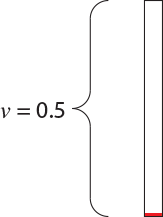in which we have a single branch of length $v = 0.5$ starting in the nucleotide $G$. How can we simulate the evolution of the site starting from the $G$ at the ancestor? The rate matrix tells us how to do this. First of all, because the current state of the process is $G$, the only relevant row of the rate matrix is the third one:

${\mathbf Q} = \{q_{ij}\} = \left( \begin{array}{cccc} \cdot & \cdot & \cdot & \cdot \\ \cdot & \cdot & \cdot & \cdot \\ 1.266 & 0.190 & -1.519 & 0.063 \\ \cdot & \cdot & \cdot & \cdot \\ \end{array} \right)$

The overall rate of change away from nucleotide $G$ is $q_{GA} + q_{GC} + q_{GT} = 1.266 + 0.190 + 0.063 = 1.519$. Equivalently, the rate of change away from nucleotide $G$ is simply $-q_{GG} = 1.519$. In a continuous-time Markov model, the waiting time between substitutions is exponentially distributed. The exact shape of the exponential distribution is determined by its rate, which is the same as the rate of the corresponding process in the ${\mathbf Q}$ matrix. For instance, if we are in state $G$, we wait an exponentially distributed amount of time with rate 1.519 until the next substitution occurs.

I generated an exponential(1.519) random variable by first generating a uniform(0,1) random number with my die. The first number it generated is $u = 0.794$. This means that the next time at which a substitution occurs is 0.152 up from the root of the tree [i.e., $t = -{1 \over 1.519} \log(0.794)$]. We can now color a portion of the branch because we know the process was in state $G$ from the root of the single-branch tree ($t=0.0$) to $t=0.152$: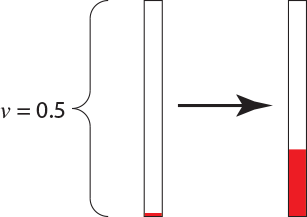The rate matrix also specifies the probabilities of a change from $G$ to the nucleotides $A$, $C$, and $T$. These probabilities are $\begin{array}{ccc} G \rightarrow A: {1.266\over 1.519}=0.833, & G \rightarrow C: {0.190\over 1.519}=0.125, & G \rightarrow T: {0.063 \over 1.519}=0.042 \\ \end{array}$ To determine what nucleotide the process changes to we would generate another uniform(0,1) random number (again called $u$). If $u$ is between 0 and 0.833, we will say that we had a change from $G$ to $A$. If the random number is between 0.833 and 0.958 we will say that we had a change from $G$ to $C$. Finally, if the random number $u$ is between 0.958 and 1.000, we will say we had a change from $G$ to $T$. The next number generated using the die was $u = 0.102$, which means the change was from $G$ to $A$. The process is now in a different state (the nucleotide $A$) and the relevant row of the rate matrix is

${\mathbf Q} = \{q_{ij}\} = \left( \begin{array}{cccc} -0.886 & 0.190 & 0.633 & 0.063 \\ \cdot & \cdot & \cdot & \cdot \\ \cdot & \cdot & \cdot & \cdot \\ \cdot & \cdot & \cdot & \cdot \\ \end{array} \right)$

We wait an exponentially distributed amount of time with parameter $\lambda = 0.886$ until the next substitution occurs. When the substitution occurs, it is to a $C$, $G$, or $T$ with probabilities ${0.190 \over 0.886} = 0.214$, ${0.633 \over 0.886} = 0.714$, and ${0.063 \over 0.886} = 0.072$, respectively.

This process of generating random and exponentially-distributed times until the next substitution occurs and then determining (randomly) what nucleotide the change is to is repeated until the process exceeds the length of the branch. The state the process is in when it passes the end of the branch is recorded. To complete the simulation on the branch, I generated another uniform random variable using the die. The number was $u = 0.371$, which means that the next substitution would occur 1.119 units above the substitution from $G \rightarrow A$. The process is in the state $A$ when it passed the end of the branch: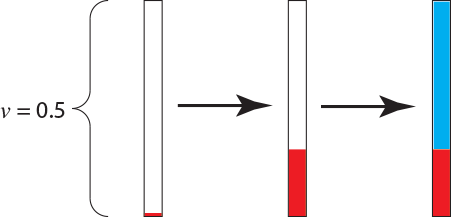The only non-random part of the entire procedure was the initial choice of the parameters. All other aspects of the simulation used a uniform random number generator and our knowledge of the rate matrix to simulate a single realization of the HKY85 process of DNA substitution.

## Simulating on a more complicated tree

Simulating on the treeis only slightly more complicated than simulating data on the single-branch tree. The steps are as follows:

• First, generate the state at the root of the tree. This step requires knowledge of the stationary probabilities for the Markov process specified by the rate matrix, ${\mathbf Q}$. The stationary distribution is the probability of capturing the process in a particular state when it has been run for a very long (technically, infinitely long) time. The stationary probabilities for the rate matrix we chose are $\pi_A = 0.4$, $\pi_C = 0.3$, $\pi_G = 0.2$, and $\pi_T = 0.1$.
• Visit each branch in turn in preorder sequence (that is, from the root to the tips of the tree). If you visit the branches in preorder sequence, you will know the state at the root of the branch.

## Pattern probabilities

The tree we simulate DNA sequence evolution on has only four tips. This means that there are a total of $4^4 = 256$ possible patterns of nucleotides we could have observed at the tips of the tree. For example, one of the possible patterns is GTTC: Species I has the nucletide G, Species II and Species III are assigned the nucleotide T, and Species IV is assigned C.

The probability of simulating any of the 256 patterns is given in the following table:

Pattern Prob. Pattern Prob. Pattern Prob. Pattern Prob.
AAAA 0.199465 AGAA 0.014711 CAAA 0.018317 CGAA 0.001490
AAAC 0.004185 AGAC 0.000725 CAAC 0.000628 CGAC 0.000210
AAAG 0.014711 AGAG 0.019868 CAAG 0.001490 CGAG 0.002878
AAAT 0.001395 AGAT 0.000242 CAAT 0.000166 CGAT 0.000048
AACA 0.009075 AGCA 0.000843 CACA 0.005277 CGCA 0.000669
AACC 0.000703 AGCC 0.000315 CACC 0.004524 CGCC 0.002262
AACG 0.000843 AGCG 0.002202 CACG 0.000669 CGCG 0.002304
AACT 0.000121 AGCT 0.000048 CACT 0.000375 CGCT 0.000188
AAGA 0.028625 AGGA 0.005985 CAGA 0.003304 CGGA 0.001065
AAGC 0.000702 AGGC 0.000755 CAGC 0.000210 CGGC 0.000209
AAGG 0.005985 AGGG 0.032738 CAGG 0.001065 CGGG 0.006655
AAGT 0.000234 AGGT 0.000252 CAGT 0.000048 CGGT 0.000059
AATA 0.003025 AGTA 0.000281 CATA 0.000959 CGTA 0.000120
AATC 0.000121 AGTC 0.000048 CATC 0.000360 CGTC 0.000180
AATG 0.000281 AGTG 0.000734 CATG 0.000120 CGTG 0.000420
AATT 0.000154 AGTT 0.000073 CATT 0.000404 CGTT 0.000202
ACAA 0.004185 ATAA 0.001395 CCAA 0.000628 CTAA 0.000166
ACAC 0.005482 ATAC 0.000350 CCAC 0.009592 CTAC 0.000415
ACAG 0.000725 ATAG 0.000242 CCAG 0.000210 CTAG 0.000048
ACAT 0.000350 ATAT 0.001594 CCAT 0.000415 CTAT 0.001214
ACCA 0.000703 ATCA 0.000121 CCCA 0.004524 CTCA 0.000375
ACCC 0.019527 ATCC 0.000752 CCCC 0.167489 CTCC 0.005866
ACCG 0.000315 ATCG 0.000048 CCCG 0.002262 CTCG 0.000188
ACCT 0.000752 ATCT 0.001546 CCCT 0.005866 CTCT 0.007452
ACGA 0.000702 ATGA 0.000234 CCGA 0.000210 CTGA 0.000048
ACGC 0.001837 ATGC 0.000116 CCGC 0.004796 CTGC 0.000208
ACGG 0.000755 ATGG 0.000252 CCGG 0.000209 CTGG 0.000059
ACGT 0.000116 ATGT 0.000535 CCGT 0.000208 CTGT 0.000607
ACTA 0.000121 ATTA 0.000154 CCTA 0.000360 CTTA 0.000404
ACTC 0.001781 ATTC 0.000517 CCTC 0.011625 CTTC 0.001716
ACTG 0.000048 ATTG 0.000073 CCTG 0.000180 CTTG 0.000202
ACTT 0.000517 ATTT 0.004711 CCTT 0.001716 CTTT 0.013873
GAAA 0.045565 GGAA 0.005060 TAAA 0.006106 TGAA 0.000497
GAAC 0.001004 GGAC 0.000453 TAAC 0.000166 TGAC 0.000048
GAAG 0.005060 GGAG 0.017648 TAAG 0.000497 TGAG 0.000959
GAAT 0.000335 GGAT 0.000151 TAAT 0.000099 TGAT 0.000038
GACA 0.002514 GGCA 0.000532 TACA 0.000959 TGCA 0.000120
GACC 0.000315 GGCC 0.000194 TACC 0.000548 TGCC 0.000274
GACG 0.000532 GGCG 0.002904 TACG 0.000120 TGCG 0.000420
GACT 0.000048 GGCT 0.000036 TACT 0.000215 TGCT 0.000108
GAGA 0.014437 GGGA 0.008240 TAGA 0.001101 TGGA 0.000355
GAGC 0.000476 GGGC 0.001251 TAGC 0.000048 TGGC 0.000059
GAGG 0.008240 GGGG 0.056794 TAGG 0.000355 TGGG 0.002218
GAGT 0.000159 GGGT 0.000417 TAGT 0.000038 TGGT 0.000030
GATA 0.000838 GGTA 0.000177 TATA 0.001119 TGTA 0.000143
GATC 0.000048 GGTC 0.000036 TATC 0.000231 TGTC 0.000116
GATG 0.000177 GGTG 0.000968 TATG 0.000143 TGTG 0.000488
GATT 0.000073 GGTT 0.000040 TATT 0.000893 TGTT 0.000447
GCAA 0.001004 GTAA 0.000335 TCAA 0.000166 TTAA 0.000099
GCAC 0.001837 GTAC 0.000116 TCAC 0.001389 TTAC 0.000240
GCAG 0.000453 GTAG 0.000151 TCAG 0.000048 TTAG 0.000038
GCAT 0.000116 GTAT 0.000535 TCAT 0.000240 TTAT 0.002009
GCCA 0.000315 GTCA 0.000048 TCCA 0.000548 TTCA 0.000215
GCCC 0.009764 GTCC 0.000376 TCCC 0.019456 TTCC 0.001275
GCCG 0.000194 GTCG 0.000036 TCCG 0.000274 TTCG 0.000108
GCCT 0.000376 GTCT 0.000773 TCCT 0.001275 TTCT 0.006924
GCGA 0.000476 GTGA 0.000159 TCGA 0.000048 TTGA 0.000038
GCGC 0.001823 GTGC 0.000117 TCGC 0.000694 TTGC 0.000120
GCGG 0.001251 GTGG 0.000417 TCGG 0.000059 TTGG 0.000030
GCGT 0.000117 GTGT 0.000530 TCGT 0.000120 TTGT 0.001005
GCTA 0.000048 GTTA 0.000073 TCTA 0.000231 TTTA 0.000893
GCTC 0.000891 GTTC 0.000258 TCTC 0.004935 TTTC 0.003240
GCTG 0.000036 GTTG 0.000040 TCTG 0.000116 TTTG 0.000447
GCTT 0.000258 GTTT 0.002355 TCTT 0.003240 TTTT 0.031522

## Exercises

Simulate a site on the four-species tree described in this lab using the rate matrix.

${\mathbf Q} = \{q_{ij}\} = \left( \begin{array}{rrrr} -0.886 & 0.190 & 0.633 & 0.063 \\ 0.253 & -0.696 & 0.127 & 0.316 \\ 1.266 & 0.190 & -1.519 & 0.063 \\ 0.253 & 0.949 & 0.127 & -1.329 \\ \end{array} \right)$

Why do we start the simulation by drawing from the stationary distribution?